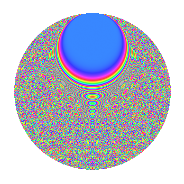# Properties

 Label 349.2.kLevel 349 Weight 2 Character orbit k Rep. character $$\chi_{349}(3,\cdot)$$ Character field $$\Q(\zeta_{174})$$ Dimension 1624 Newforms 1 Sturm bound 58 Trace bound 0

# Related objects

## Defining parameters

 Level: $$N$$ = $$349$$ Weight: $$k$$ = $$2$$ Character orbit: $$[\chi]$$ = 349.k (of order $$174$$ and degree $$56$$) Character conductor: $$\operatorname{cond}(\chi)$$ = $$349$$ Character field: $$\Q(\zeta_{174})$$ Newforms: $$1$$ Sturm bound: $$58$$ Trace bound: $$0$$

## Dimensions

The following table gives the dimensions of various subspaces of $$M_{2}(349, [\chi])$$.

Total New Old
Modular forms 1736 1736 0
Cusp forms 1624 1624 0
Eisenstein series 112 112 0

## Trace form

 $$1624q - 55q^{2} - 58q^{3} - 85q^{4} - 60q^{5} - 58q^{6} - 58q^{7} - 145q^{8} - 29q^{9} + O(q^{10})$$ $$1624q - 55q^{2} - 58q^{3} - 85q^{4} - 60q^{5} - 58q^{6} - 58q^{7} - 145q^{8} - 29q^{9} - 58q^{10} - 58q^{11} - 57q^{12} - 55q^{13} - 48q^{14} - 59q^{15} - 29q^{16} - 48q^{17} + 43q^{18} + 67q^{19} - 58q^{20} + 58q^{21} - 55q^{22} - 41q^{23} - 39q^{24} - 29q^{25} - 112q^{26} - 76q^{27} - 58q^{28} - 56q^{29} - 67q^{30} - 90q^{31} - 67q^{32} - 46q^{33} + 5q^{34} - 82q^{36} + 45q^{37} - 58q^{38} - 58q^{39} - 112q^{40} + 10q^{41} + 305q^{42} - 13q^{43} + 40q^{44} - 56q^{45} - 85q^{46} - 58q^{47} - 122q^{48} - 93q^{49} - 441q^{50} - 160q^{51} + 58q^{52} - 58q^{53} - 85q^{54} + 34q^{55} - 69q^{56} - 29q^{57} - 58q^{58} - 40q^{59} + 522q^{60} + 87q^{61} - 49q^{62} - 37q^{63} + 335q^{64} - 58q^{65} - 565q^{66} - 116q^{67} - 58q^{68} + 16q^{69} - 98q^{70} - 34q^{71} + 44q^{72} - 52q^{73} - 204q^{74} - 95q^{76} - 54q^{77} + 439q^{78} - 58q^{79} - 192q^{80} - 209q^{81} - 79q^{82} + 162q^{83} + 330q^{84} - 192q^{85} + 126q^{86} - 190q^{87} - 8q^{88} + 345q^{89} - 46q^{90} - 30q^{91} + 62q^{92} - 89q^{93} - 87q^{94} - 350q^{95} + 990q^{96} - 43q^{97} - 464q^{98} - 97q^{99} + O(q^{100})$$

## Decomposition of $$S_{2}^{\mathrm{new}}(349, [\chi])$$ into irreducible Hecke orbits

Label Dim. $$A$$ Field CM Traces $q$-expansion
$$a_2$$ $$a_3$$ $$a_5$$ $$a_7$$
349.2.k.a $$1624$$ $$2.787$$ None $$-55$$ $$-58$$ $$-60$$ $$-58$$